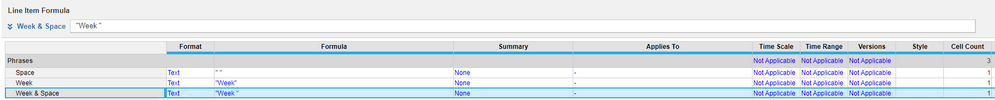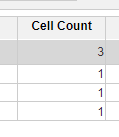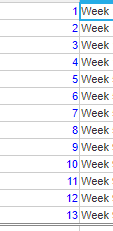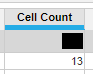# Reducing Text Calculationsedited December 2022

## In short:

1) Create a module that doesn't have any dimensions applied to it
2) Every time you need to use a static text value create a new line item that is formatted as text, with the formula being that word or phrase. In this example " Week" will be used in this example.

3) Reference this line item inside of the calculations that need it.

Benefits: you are calculating once, versus however many line item members there are! This leads to better performance, fewer redundancies, and a 1 stop shop for all non-dynamic text.

## The long version and further details:

Reducing calculations in Anaplan improves performance, and I am not referring to reducing line items. I am talking about the pieces of each formula in the line items. Anaplan’s engine is designed primarily to complete tasks that are boolean, followed by numerical, like most computers. When you leverage text, Anaplan must work significantly harder to complete the created equation. Text calculations are necessary for many tasks, so when you do need to leverage them, being efficient by reducing the number of times that Anaplan needs to calculate pieces of, or at all, will return in an improvement in performance. One of those methods is to reduce dimensions. An easy way to reduce the number of dimensions is to create a reference module without lists in the applies to. This way, the line items within this module only have a cell count of one.

In this example, our end goal is to add the term “Week “ into a line item, for all list members. The module does not require a summary, so this is assumed to function as a flat list. With summaries enabled, the calculations get more complex on how Anaplan handles it.

For consistency sake and clarity, I built a reference module called “Phrases” which does not have any lists in the applies to and does not use Time or Versions. In this instance, I have two commonly used phrases in the model, "Week" and space. I also added another line item with the specific combined phrase, as the target example module requires them to be together. Concatenation is the hardest process on Anaplan's calculation engine for a single dimension. Thus the goal is to have any text calculations that are applied to more than one cell calculated in the Phrases module, shown below.You can reduce the number of text calculations to one reference, rather than X number of calculations, in this instance, there are 13 list members, which you can see by the cell count. The following is done by applying the equation which references the Phrases module:## This results in:In this example, if the line item above were to be written as ..."Week" & " " &... the Hyperblock would be performing the functions of the formula from the two sets of the ellipsis(...), in addition to 3 extra calculations per list member and each other dimension such as Time and Versions. In addition, if the formula summary type is not set to none, additional calculation per intersection at the higher levels will also occur!

In this specific example, we are using close to a flat list, with 1 parent value; summary is turned off. The list has 13 members. This means, if using the long form formula; ..."Week" & " " &... , the Hyperblock is completing 13 text + 13 concatenations + 13 text + 13 concatenations plus any additional functions the other portions of the formula to the left or right of the ellipsis. That is a minimum of 52 calculations for this line item, not including the ellipsis portion of the formula!

If you apply the method that is described in the first half of the article; Phrases.'Week & Space' &, the calculations will be reduced to 1 reference + 13 concatenations and any additional calculations beyond the ellipsis, bring it down to only 14! This results in a savings of 38 calculations and using the Hyperblock as effectively as possible.

Note, this is a simple example, and the difference can be significantly more depending on the dimensionality, design, and size of your model.

## To recap

Normal method: 13 text calculations per change impacting the line item AND during model launch

Described Method: 3 text calculations during model launch and 1 reference calculation per change impacting the line item AND during model launch.

•